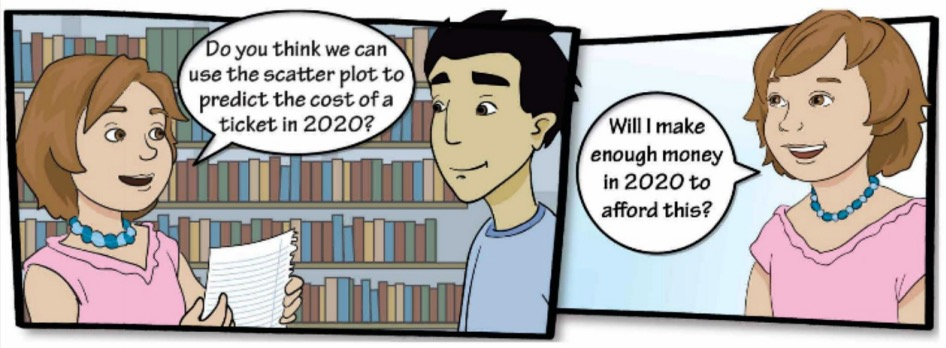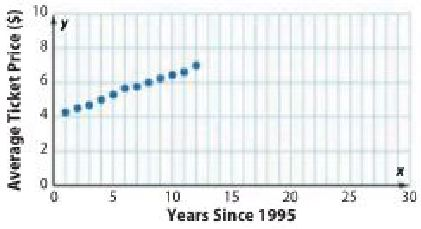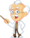Homework Explained - Math Practice 101Dear guest, you are not a registered member. As a guest, you only have read-only access to our books, tests and other practice materials.

As a registered member you can:

 Question 4 Model with Mathematics Refer to the graphical novel frame below for Exercises a-b.a. The scatter plot shows the average ticket prices since 1995. Draw a line that best represents the data in your scatter plot.Type below:(show solution) Question 4 b. Write an equation in slope-intercept form for the line of best fit. Make a conjecture about the cost of a movie ticket in 2020. Type below:(show solution) H.O.T. Problems Higher Order Thinking Question 5 Use Math Tools Use a newspaper or the Internet to find a scatter plot that consists of at least seven data points. Tape the scatter plot to your book. Draw a line of best fit and write an equation for the line. Type below:(show solution) Question 6 Persevere with Problems Describe or draw a scatter plot where a line of fit does not model the data. Explain your reasoning to a classmate. Type below:(show solution) Question 7 Justify Conclusions Determine whether each statement is always, sometimes, or never true for data with a positive association. Justify your response. a. The statement of the line of best fit is positive. Type below:(show solution) Question 7 b. The y-intercept is positive. Type below:(show solution)